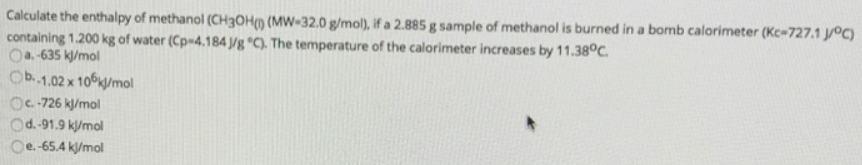# Problem: Calculate the enthalpy of methanol (CH3OH(l) (MW = 32.0 g/mol), if a 2.885 g sample of methanol is burned in a bomb calorimeter (Kc = 727.1 J/°C) containing 1.200 kg of water (Cp = 4.184 J/°C). The temperature of the calorimeter increases by 11.38°C. a. 635 kJ/molb. -1.02 x 106 kJ/mol c. -726 kJ/mol  d. -91.9 kJ/mol  e. -65.4 kJ/mol

###### FREE Expert Solution
83% (151 ratings)###### Problem Details

Calculate the enthalpy of methanol (CH3OH(l) (MW = 32.0 g/mol), if a 2.885 g sample of methanol is burned in a bomb calorimeter (Kc = 727.1 J/°C) containing 1.200 kg of water (Cp = 4.184 J/°C). The temperature of the calorimeter increases by 11.38°C.

a. 635 kJ/mol

b. -1.02 x 106 kJ/mol

c. -726 kJ/mol

d. -91.9 kJ/mol

e. -65.4 kJ/mol